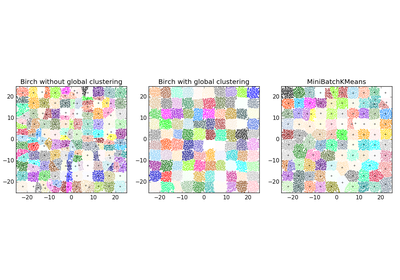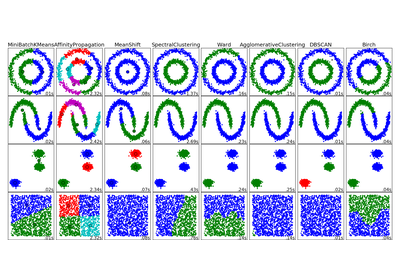# `sklearn.cluster`.Birch¶

class `sklearn.cluster.``Birch`(threshold=0.5, branching_factor=50, n_clusters=3, compute_labels=True, copy=True)[source]

Implements the Birch clustering algorithm.

Every new sample is inserted into the root of the Clustering Feature Tree. It is then clubbed together with the subcluster that has the centroid closest to the new sample. This is done recursively till it ends up at the subcluster of the leaf of the tree has the closest centroid.

Read more in the User Guide.

Parameters: threshold : float, default 0.5 The radius of the subcluster obtained by merging a new sample and the closest subcluster should be lesser than the threshold. Otherwise a new subcluster is started. branching_factor : int, default 50 Maximum number of CF subclusters in each node. If a new samples enters such that the number of subclusters exceed the branching_factor then the node has to be split. The corresponding parent also has to be split and if the number of subclusters in the parent is greater than the branching factor, then it has to be split recursively. n_clusters : int, instance of sklearn.cluster model, default 3 Number of clusters after the final clustering step, which treats the subclusters from the leaves as new samples. If None, this final clustering step is not performed and the subclusters are returned as they are. If a model is provided, the model is fit treating the subclusters as new samples and the initial data is mapped to the label of the closest subcluster. If an int is provided, the model fit is AgglomerativeClustering with n_clusters set to the int. compute_labels : bool, default True Whether or not to compute labels for each fit. copy : bool, default True Whether or not to make a copy of the given data. If set to False, the initial data will be overwritten. root_ : _CFNode Root of the CFTree. dummy_leaf_ : _CFNode Start pointer to all the leaves. subcluster_centers_ : ndarray, Centroids of all subclusters read directly from the leaves. subcluster_labels_ : ndarray, Labels assigned to the centroids of the subclusters after they are clustered globally. labels_ : ndarray, shape (n_samples,) Array of labels assigned to the input data. if partial_fit is used instead of fit, they are assigned to the last batch of data.

References

Examples

```>>> from sklearn.cluster import Birch
>>> X = [[0, 1], [0.3, 1], [-0.3, 1], [0, -1], [0.3, -1], [-0.3, -1]]
>>> brc = Birch(branching_factor=50, n_clusters=None, threshold=0.5,
... compute_labels=True)
>>> brc.fit(X)
Birch(branching_factor=50, compute_labels=True, copy=True, n_clusters=None,
threshold=0.5)
>>> brc.predict(X)
array([0, 0, 0, 1, 1, 1])
```

Methods

 `fit`(X[, y]) Build a CF Tree for the input data. `fit_predict`(X[, y]) Performs clustering on X and returns cluster labels. `fit_transform`(X[, y]) Fit to data, then transform it. `get_params`([deep]) Get parameters for this estimator. `partial_fit`([X, y]) Online learning. `predict`(X) Predict data using the `centroids_` of subclusters. `set_params`(\*\*params) Set the parameters of this estimator. `transform`(X[, y]) Transform X into subcluster centroids dimension.
`__init__`(threshold=0.5, branching_factor=50, n_clusters=3, compute_labels=True, copy=True)[source]
`fit`(X, y=None)[source]

Build a CF Tree for the input data.

Parameters: X : {array-like, sparse matrix}, shape (n_samples, n_features) Input data.
`fit_predict`(X, y=None)[source]

Performs clustering on X and returns cluster labels.

Parameters: X : ndarray, shape (n_samples, n_features) Input data. y : ndarray, shape (n_samples,) cluster labels
`fit_transform`(X, y=None, **fit_params)[source]

Fit to data, then transform it.

Fits transformer to X and y with optional parameters fit_params and returns a transformed version of X.

Parameters: X : numpy array of shape [n_samples, n_features] Training set. y : numpy array of shape [n_samples] Target values. X_new : numpy array of shape [n_samples, n_features_new] Transformed array.
`get_params`(deep=True)[source]

Get parameters for this estimator.

Parameters: deep : boolean, optional If True, will return the parameters for this estimator and contained subobjects that are estimators. params : mapping of string to any Parameter names mapped to their values.
`partial_fit`(X=None, y=None)[source]

Online learning. Prevents rebuilding of CFTree from scratch.

Parameters: X : {array-like, sparse matrix}, shape (n_samples, n_features), None Input data. If X is not provided, only the global clustering step is done.
`predict`(X)[source]

Predict data using the `centroids_` of subclusters.

Avoid computation of the row norms of X.

Parameters: X : {array-like, sparse matrix}, shape (n_samples, n_features) Input data. labels: ndarray, shape(n_samples) : Labelled data.
`set_params`(**params)[source]

Set the parameters of this estimator.

The method works on simple estimators as well as on nested objects (such as pipelines). The latter have parameters of the form `<component>__<parameter>` so that it’s possible to update each component of a nested object.

Returns: self :
`transform`(X, y=None)[source]

Transform X into subcluster centroids dimension.

Each dimension represents the distance from the sample point to each cluster centroid.

Parameters: X : {array-like, sparse matrix}, shape (n_samples, n_features) Input data. X_trans : {array-like, sparse matrix}, shape (n_samples, n_clusters) Transformed data.

## Examples using `sklearn.cluster.Birch`¶Compare BIRCH and MiniBatchKMeansComparing different clustering algorithms on toy datasets